# Isosceles Triangles

Go back to  'Triangles-Quadrilaterals'

An isosceles triangle is a triangle in which two sides are equal. In the following figure, ∆ABC is isosceles, with AB = AC: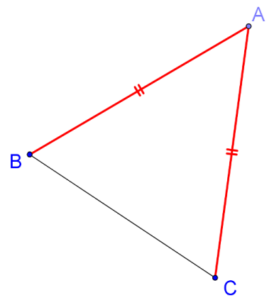The angle between the equal sides (in this case, ∠A) is termed the vertical angle of the isosceles triangle, while the side opposite the vertical angle (in this case, BC) is termed the base of the isosceles triangle. We will now discuss some important facts related to isosceles triangles.

Fact 1: The two non-vertical angles (the angles opposite the equal sides) in any isosceles triangle are equal.

Proof: Let ∆ABC be an isosceles triangle with AB = AC. We will compare ∆ABC with itself. In the following figure, on the left – you have ∆ABC, while on the right – you have a flipped version of ∆ABC, which we can call ∆ACB.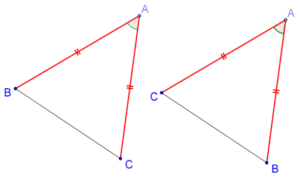Now, compare the two triangles:

1. AB = AC
2. AC = AB
3. ∠A = ∠A (common included angle)

Thus, ∆ABC ≡ ∆ACB, which means that the corresponding angles are equal. Hence, ∠B = ∠C, which proves that the angles opposite the equal sides in an isosceles triangle are equal.

Fact 2: If two angles are equal in a triangle, then it is isosceles, with the sides opposite the equal angles being equal.

Proof: Suppose that in ∆ABC, ∠B = ∠C. We have to prove that AB = AC. Once again, we compare ∆ABC with (a flipped version of) itself. In the following figure, we are comparing ∆ABC and ∆ACB: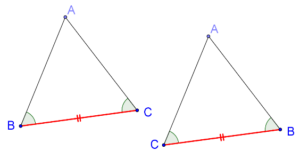We note that:

1. BC = CB (common base)

2. ∠B = ∠C

3. ∠C = ∠B

Thus, ∆ABC ≡ ∆ACB by the ASA criterion. This means that AB = AC, and hence our proof is complete.

Alternatively, we could have drawn the angle bisector through the vertex A, as shown below: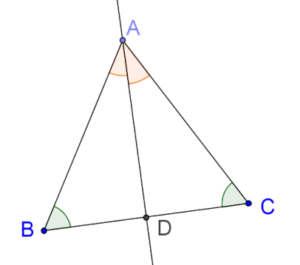We now compare ∆ABD and ∆ACD:

2. ∠B = ∠C
3. ∠BAD = ∠CAD (by our construction)

Thus, ∆ABD ≡ ∆ACD, which means that AB = AC.

Fact 3: In any isosceles triangle, the median through the vertical angle is perpendicular to the base, and bisects the vertical angle.

Proof: Recall that the median through a vertex bisects the opposite side. Suppose that ABC is an isosceles triangle, in which the median through A bisects BC at D: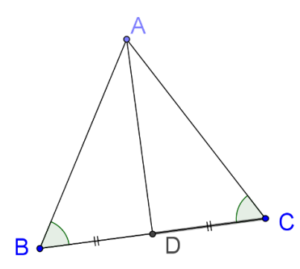We have to prove that AD is perpendicular to BC. We compare ∆ABD with ∆ACD:

1. AB = AC (because ∆ABC is isosceles)

2. BD = CD (because AD is the median to BC)

3. ∠B = ∠C (we have proved that this follows from the fact that AB = AC)

Thus, by the SAS criterion, ∆ABD ≡ ∆ACD. This means that:

• ∠BDA = ∠CDA = ½ × 180° = 90°. In other words, the median AD is perpendicular to the base BC.

• ∠BAD = ∠CAD In other words, the median AD bisects ∠A, the vertical angle.